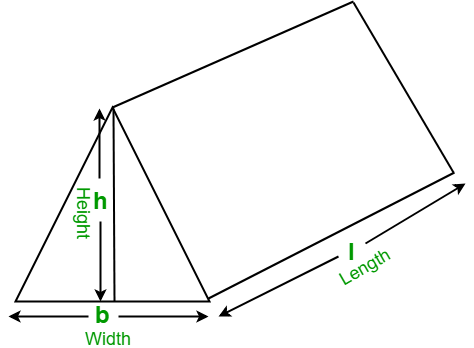# Program to find the Volume of a Triangular Prism

Given the length, width, and height of a triangular prism, the task is to find the volume of the triangular prism.
Examples:

```Input:  l = 18, b = 12, h = 9
Output: Volume of triangular prism: 972

Input: l = 10, b = 8, h = 6
Output: Volume of triangular prism: 240
```

## Recommended: Please try your approach on {IDE} first, before moving on to the solution.

In mathematics, a triangular prism is a three-dimensional solid shape with two identical ends connected by equal parallel lines. The triangular prism contains 5 faces, 9 edges, and 6 vertices.Formula to find the volume of triangular prism:

`Volume = ( l * b * h ) / 2 `

## C++

 `// CPP program to find the volume ` `// of the triangular prism ` `#include ` `using` `namespace` `std; ` ` `  `// function to find the Volume ` `// of triangular prism ` `float` `findVolume(``float` `l, ``float` `b, ``float` `h) ` `{ ` `    ``// formula to find Volume ` `    ``float` `volume = (l * b * h) / 2; ` ` `  `    ``return` `volume; ` `} ` ` `  `// Driver Code ` `int` `main() ` `{ ` `    ``float` `l = 18, b = 12, h = 9; ` ` `  `    ``// function calling ` `    ``cout << ``"Volume of triangular prism: "` `         ``<< findVolume(l, b, h); ` ` `  `    ``return` `0; ` `} `

## Java

 `// Java program to find the volume ` `// of the triangular prism ` `import` `java.io.*; ` ` `  `class` `GFG { ` ` `  `    ``// function to find the Volume ` `    ``// of triangular prism ` `    ``static` `float` `findVolume(``float` `l, ``float` `b, ``float` `h) ` `    ``{ ` `        ``// formula to find Volume ` `        ``float` `volume = (l * b * h) / ``2``; ` ` `  `        ``return` `volume; ` `    ``} ` ` `  `    ``// Driver code ` `    ``public` `static` `void` `main(String[] args) ` `    ``{ ` `        ``float` `l = ``18``, b = ``12``, h = ``9``; ` ` `  `        ``// function calling ` `        ``System.out.println(``"Volume of triangular prism: "` `                           ``+ findVolume(l, b, h)); ` `    ``} ` `} `

## Python3

 `# Python3 program to find the volume ` `# of the triangular prism ` ` `  `# function to find the Volume  ` `# of triangular prism ` `def` `findVolume(l, b, h) : ` ` `  `    ``# formula to find Volume ` `    ``return` `((l ``*` `b ``*` `h) ``/` `2``) ` ` `  `# Driver Code ` `l ``=` `18` `b ``=` `12` `h ``=` `9` `     `  `# function calling ` `print``(``"Volume of triangular prism: "``, ` `                ``findVolume(l, b, h)) `

## C#

 `// C# program to find the volume ` `// of the triangular prism ` `using` `System; ` ` `  `class` `GFG { ` ` `  `    ``// function to find the Volume ` `    ``// of triangular prism ` `    ``static` `float` `findVolume(``float` `l, ``float` `b, ``float` `h) ` `    ``{ ` `        ``// formula to find Volume ` `        ``float` `volume = (l * b * h) / 2; ` ` `  `        ``return` `volume; ` `    ``} ` ` `  `    ``// Driver code ` `    ``static` `public` `void` `Main() ` `    ``{ ` `        ``float` `l = 18, b = 12, h = 9; ` ` `  `        ``// function calling ` `        ``Console.WriteLine(``"Volume of triangular prism: "` `                          ``+ findVolume(l, b, h)); ` `    ``} ` `} `

## PHP

 ` `

Output:

```Volume of triangular prism: 972
```

Attention reader! Don’t stop learning now. Get hold of all the important DSA concepts with the DSA Self Paced Course at a student-friendly price and become industry ready.

My Personal Notes arrow_drop_upCheck out this Author's contributed articles.

If you like GeeksforGeeks and would like to contribute, you can also write an article using contribute.geeksforgeeks.org or mail your article to contribute@geeksforgeeks.org. See your article appearing on the GeeksforGeeks main page and help other Geeks.

Please Improve this article if you find anything incorrect by clicking on the "Improve Article" button below.

Article Tags :
Practice Tags :

Be the First to upvote.

Please write to us at contribute@geeksforgeeks.org to report any issue with the above content.